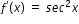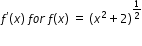# MA.912.C.2.4

Apply the rules for finding derivatives of constants, sums, products, quotients and the Chain Rule to solve problems with functions limited to algebraic, trigonometric, inverse trigonometric, logarithmic and exponential.

### Examples

Example: Findfor the function y=ln x

Example: Show that the derivative of f(x)=tan x isusing the quotient rule for derivatives.

Example: Find.

### Clarifications

Clarification 1: Special cases of rules include a constant multiple of a function and the power of a function.
General Information
Subject Area: Mathematics (B.E.S.T.)
Strand: Calculus
Status: State Board Approved

## Related Courses

This benchmark is part of these courses.
1202300: Calculus Honors (Specifically in versions: 2014 - 2015, 2015 - 2022, 2022 and beyond (current))

## Related Access Points

Alternate version of this benchmark for students with significant cognitive disabilities.

## Related Resources

Vetted resources educators can use to teach the concepts and skills in this benchmark.

## Tutorials

Using the Product Rule and the Chain Rule:

In this video we will use the chain rule and the product rule together to find a derivative of a composite function.

Type: Tutorial

The Product Rule for Derivatives:

In this video will will apply the product rule to find the derivative of two functions.

Type: Tutorial

Product Rule for More Than Two Functions:

In this video, we will use the product rule to find the derivative of the product of three functions.

Type: Tutorial

Derivative of Log with Arbitrary Base:

In this video, we will find the derivative of a log with an arbitrary base.

Type: Tutorial

Chain Rule for Derivative of 2^x:

Here we will see how the chain rule is used to find the derivative of a logarithmic function.

Type: Tutorial

Chain Rule Introduction:

This video is an introduction on how to apply the chain rule to find the derivative of a composite function.

Type: Tutorial

Chain Rule Definition and Example:

In this video we will define the chain rule and use it to find the derivative of a function.

Type: Tutorial

Chain Rule Example Using Visual Information:

In this video we will analyze the graph of a function and its tangent line, then use the chain rule to find the value of the derivative at that point.

Type: Tutorial

Chain Rule Example Using Visual Function Definitions:

We will use the chain rule to find the value of a composite function at a given point, given the graphs of the two composing functions.

Type: Tutorial

## Student Resources

Vetted resources students can use to learn the concepts and skills in this benchmark.

## Tutorials

Using the Product Rule and the Chain Rule:

In this video we will use the chain rule and the product rule together to find a derivative of a composite function.

Type: Tutorial

The Product Rule for Derivatives:

In this video will will apply the product rule to find the derivative of two functions.

Type: Tutorial

Product Rule for More Than Two Functions:

In this video, we will use the product rule to find the derivative of the product of three functions.

Type: Tutorial

Derivative of Log with Arbitrary Base:

In this video, we will find the derivative of a log with an arbitrary base.

Type: Tutorial

Chain Rule for Derivative of 2^x:

Here we will see how the chain rule is used to find the derivative of a logarithmic function.

Type: Tutorial

Chain Rule Introduction:

This video is an introduction on how to apply the chain rule to find the derivative of a composite function.

Type: Tutorial

Chain Rule Definition and Example:

In this video we will define the chain rule and use it to find the derivative of a function.

Type: Tutorial

Chain Rule Example Using Visual Information:

In this video we will analyze the graph of a function and its tangent line, then use the chain rule to find the value of the derivative at that point.

Type: Tutorial

Chain Rule Example Using Visual Function Definitions:

We will use the chain rule to find the value of a composite function at a given point, given the graphs of the two composing functions.

Type: Tutorial

## Parent Resources

Vetted resources caregivers can use to help students learn the concepts and skills in this benchmark.